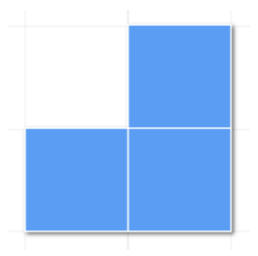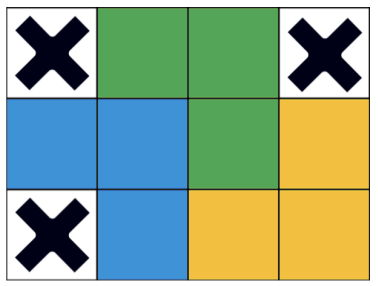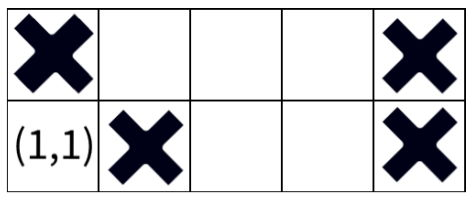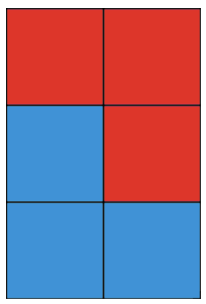시간 제한메모리 제한제출정답맞은 사람정답 비율
8 초 512 MB111100.000%

## 문제

Luka stumbled upon a rectangular board of height $H$ and width $W$ divided into $W \times H$ unit squares. He quickly noticed that exactly $K$ of those unit squares are missing.

Interestingly enough, Luka just happens to have an infinite supply of L-shaped triominoes. Is it possible to tile the given board using these triominoes?We consider the board to be correctly tiled if each unit square of the board is covered by a triomino square. Additionally, triominoes must not cover any of the missing squares, and should not overlap or stick out of the board. Of course, triominoes can be arbitrarily rotated by multiples of 90 degrees.

## 입력

The first line contains three integers $W$, $H$ and $K$ ($0 \le K \le W \cdot H$) from the task description.

The $i$-th of the next $K$ lines contains two integers $x_i$ ($1 \le x_i \le W$) and $y_i$ ($1 \le y_i \le H$), representing the coordinates of the $i$-th missing square. The given missing squares are pairwise distinct.

## 출력

If Luka can successfully tile the given board, output "YES" in a single line. Otherwise, output "NO" in a single line.

## 서브태스크

번호 배점 제한
1 10

$2 \le W \le 13$, $2 \le H \le 1\,000$, $K \le 250$

2 7

$2 \le W \le 13$, $2 \le H \le 10^9$, $K = 0$

3 11

$2 \le W \le 3$, $2 \le H \le 10^9$, $K \le 250$

4 17

$4 \le W \le 6$, $2 \le H \le 10^9$, $K \le 250$

5 35

$7 \le W \le 13$, $2 \le H \le 10^9$, $K \le 250$

6 20

$2 \le W \le 13$, $2 \le H \le 10^9$, $K \le 250$

## 예제 입력 1

4 3 3
1 1
1 3
4 3


## 예제 출력 1

YES## 예제 입력 2

5 2 4
1 2
2 1
5 1
5 2


## 예제 출력 2

NO


Luka cannot place a valid triomino which tiles square (1, 1).## 예제 입력 3

2 3 0


## 예제 출력 3

YES## 채점 및 기타 정보

• 예제는 채점하지 않는다.